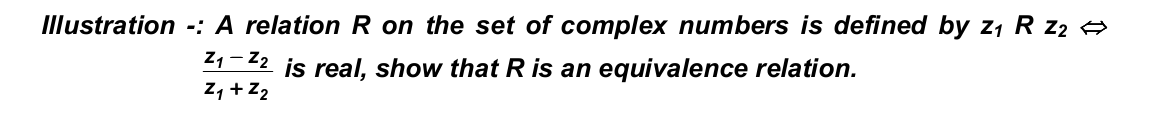Equivalence Relation:

A relation R in a set A is called an equivalence relation if

1. R is reflexive i.e., ≤ a, a) ∈ R, ” a ∈ A
2. R is symmetric i.e., ≤ a, b) ∈ R ⇒ ≤ b, a) ∈ R
3. R is transitive i.e., ≤ a, b), ≤ b, c) ∈ R ⇒ ≤ a, c) ∈R

The equivalence relation is usually denoted by the symbol ~.

Equivalence Classes of an Equivalence Relation:

Let R be equivalence relation in A ≤ ≠ ϕ). Let a ∈ A.

Then the equivalence class of a denoted by [a] or {} is defined as the set of all those points of A which are related to a under the relation R. Thus [a] = {x : x ∈ A, x R a}

It is easy to see that

1. b ∈ [a] ⇒ a ∈ [b]
2. b ∈ [a] ⇒ [a] = [b]
3. Two equivalence classes are either disjoint of identical.

as an example we consider a very important relation

x º y ≤ mod n) iff n divides ≤ x –y),  is fixed positive integer. Consider n = 5 then
 = {x : x º 0≤ mod 5)} = {5p : p ∈ z} = {0, ±5, ±10, ±15,….}
 = {x : x º 1≤ mod 5)} = {x : x –1 = 5k, k ∈ z} = {5k + 1: k ∈ z} = {1, 6, 11, …., –4, –9,….}
one can easily see that there are only 5 distinct equivalence classes viz. , , ,  and  when n = 5.

Illustration -:

N is the set of natural numbers. The relation R is defined on N x N as follows:
≤ a, b) R ≤ c, d) ↔ a + d = b + c
Prove that R is equivalence relation.

Solution:

i) ≤ a, b) R ≤ a, b) ↔ a + b = b + a
\ R is reflexive.

ii) ≤ a, b) R ≤ c, d) ⇒ a + d = b + c
⇒ c + b = d + a
⇒ ≤ c, d) R ≤ a, b)
\ R is symmetric.

Now iii) ≤ a, b) R ≤ c, d) and ≤ c, d) R ≤ e, f) ⇒ a + d = b + c & c + f = d + e
⇒ a + d + c + f = b + c + d + e
⇒ a + f = b + e ⇒ ≤ a, b) R ≤ e, f)
\ R is transitive. Thus R is an equivalence relation on N x N.